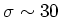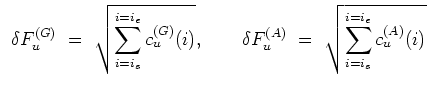Next: Durations Up: Total Counts Previous: Total Counts   Contents

#### Error Estimation

The errors on the total counts of a GRB are computed simply by propagating the errors on the single background subtracted bins. Therefore, the issue concerns the way the errors on the single bins of the background subtracted light curves have been calculated.

The error calculation is based on the following assumption: the mean systematic error owing to the background fit polynomial can be neglected, when compared with the statistical fluctuations. Since the typical mean count rate varies in the range from 800-1200 counts/s, it turns out thatcounts/s; we confidently estimate that the error introduced within the background subtraction is dominated by the statistical uncertainties. Therefore, from eq., the only term contributing to the uncertainties on the total counts estimates is the measured counts of every bin, according to the eq..(42)

Cristiano Guidorzi 2003-07-31